### Question 14. Solve the following problems: Equal heat is given to two objects A and B of mass 1 g. Temperature of A increases by 3oC and B by 5oC. Which object has more specific heat? And by what factor?

Question 14.

Solve the following problems:

Equal heat is given to two objects A and B of mass 1 g. Temperature of A increases by 3oC and B by 5oC. Which object has more specific heat? And by what factor?

Answer:

Specific heat is the amount of heat per unit mass required to raise the temperature by one degree Celsius.

It is given by the formula:

Q = mcΔT

Or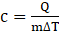Where, Q is the amount of heat added.

T is the temperature.

“c” is the specific heat

“m” is the mass

Now, let say, two objects A and B.

Then, the ratio of the specific heats of two objects is given as: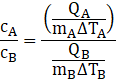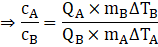As per the question, equal heat is given to two objects A and B of mass 1 g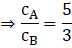Or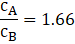Clearly, it shows that the specific heat of A is greater than B with a factor 1.66.